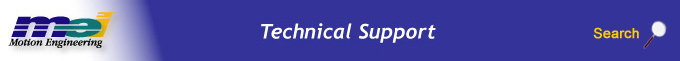.

Overview of SynqNet I/O for the MPX

## Modules/Slices/Segments Terminology

Modules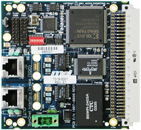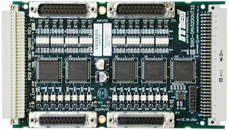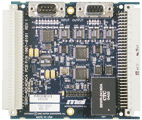SQID DIN32DOUT32 ADC4DAC4

Slices
A Slice network adaptor is expanded with slices.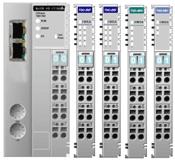Segments
The software uses the same MPI functions to access I/O on both slices or modules. The software uses the generic term "segment" for slices or modules, depending on the type of the SynqNet I/O node.

## I/O Example Network

The following diagram shows how a Slice I/O, SynqNet I/O, and RMB node could be connected. The first node, Node 0, is a Slice I/O node with three slices attached: two digital input slices and one digital output slice. The second node, Node 1, is a SynqNet I/O node with a SQID network adaptor with two modules attached, a DIN32DOUT32 and an ADC4DAC4. The third node, Node 2, is an RMB-10V2 node, this node has four analog inputs that are not associated with any motion, and these analog inputs are accessed with the same functions as the other two node types.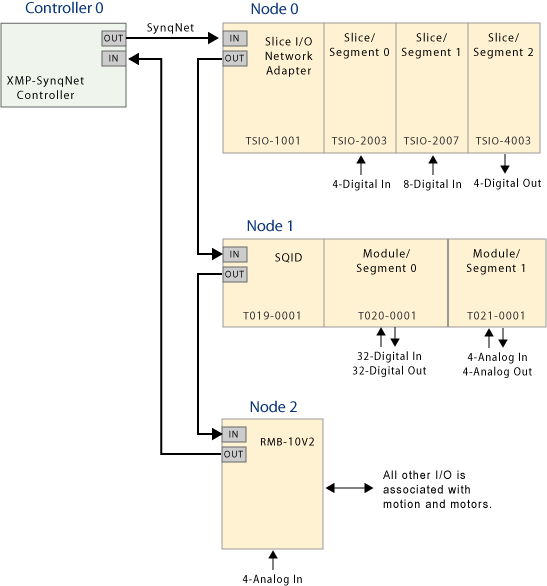## What I/O does a node support?

To determine if a node supports I/O and how much I/O is supported, you can use the SqNode.DigitalInCount, SqNode.DigitalOutCount, SqNode.AnalogInCount, and SqNode.DigitalOutCount properties.

The SqNode.SegmentCount property returns the number of segments present on a node.

The following MPX code shows how to read the number of supported digital inputs on a node 0:

## Visual Basic

 ```Dim numberOfDigitalInputs As Int32 ``` numberOfDigitalInputs =controller.SynqNet(0).Node(0).DigitalInCount

## C#

 ```int numberOfDigitalInputs; numberOfDigitalInputs = controller.SynqNet.Node.DigitalInCount; ```

The SqNode properties the nodes in the example network would be:

 Node 0 Node 1 Node 2 DigitalInCount 12 32 0 DigitalOutCount 4 32 0 AnalogInCount 0 4 4 AnalogOutCount 0 4 0 SegmentCount 3 2 0

## What Information is Available About Each I/O Segment?

The I/O on each node is comprised of a number of segments. To access information about these segments, one must use the SqNodeSegment object. One can obtain access to an SqNodeSegment object through the Segment property of the SqNode object.

## Visual Basic

 ```Dim segmentA As MPX.SqNodeSegment segmentA = controller.SynqNet(0).Node(2).Segment(0)```

## C#

 ```MPX.SqNodeSegment segmentA; segmentA = controller.SynqNet.Node.Segment;```

The SqNodeSegment object has its own DigitalInCount, DigitalOutCount, AnalogInCount, and AnalogOutCount properties. These tell how much I/O is available on the segment.

The SegmentId, Option, SerialNumber, Model, Version, and ManufacturerData properties provide additional production data.

The SegmentId property provides a unique number to identify the kind of segment. For SynqNet I/O modules:

 Type Code PN Description &H C0 FE C0 01 & T020-0001 DIN32DOUT32 &H C0 FE C0 01 & T022-0001 ADC4DAC4

These properties have the following values for each of the slices in the example network:

 Node 0 Node 1 Segment 0 Segment 1 Segment 2 Segment 0 Slice 1 Id &H00030041& &H00070041& &H000E0081& &HC0FEC001& &HC0FEC002& Option 0 0 0 0 0 SerialNumber &H9008& &H902A& &H4503& &H480012& &H480037& Model TSIO-2003 TSIO-2007 TSIO-4003 T021-0001 T021-0001 DigitalInCount 4 8 0 32 0 DigitalOutCount 0 0 4 32 0 AnalogInCount 0 0 0 0 4 AnalogOutCount 0 0 0 0 4 Version 5 5 5 0 0 ManufacturerData "" "" "" "" ""

Since Node 2 is an RMB-10V2 and does not have any slices, attempting to access a child SqNodeSegment object from SqNode(2) will generate an error.

The following MPX Visual Basic code shows how to read the number of digital inputs on Slice 1 of Node 0.

 Dim SegmentA As MPXCtl.SqNodeSegment Dim x as Long Set SegmentA = Controller.SqNode(2).Segment(0) x = SegmentA.DigitalInCount;

## Accessing Digital Data

The following digital I/O members allow single or multiple digital input or output bits on a node or segment on a node to be accessed.

SqNode.DigitalInGet
SqNode.DigitalInBit
SqNode.DigitalOutGet
SqNode.DigitalOutSet
SqNode.DigitalOutBit

Addressing I/O bits relative to each segment:

SqNodeSegment.DigitalInGet
SqNodeSegment.DigitalInBit
SqNodeSegment.DigitalOutGet
SqNodeSegment.DigitalOutSet
SqNodeSegment.DigitalOutBit

The following MPX code will get the current state of digital inputs 3, 4, and 5 of Node 0. In the example network, these bits spread over segments 0 and 1:

## Visual Basic

 ```Dim x() As Boolean x = controller.SynqNet(0).Node(0).DigitalInGet(3, 3) ```

## C#

 `bool x[] = controller.SynqNet.Node.DigitalInGet(3, 3);`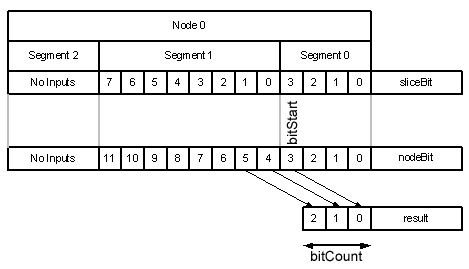The following MPX code will get the current state of all the digital inputs on segment 1 of node 0:

## Visual Basic

 ```Dim segment As Mpx.SqNodeSegment Dim x() As Boolean segment = controller.SynqNet(0).Node(0).Segment(1) x = segment.DigitalInGet(0, segment.DigitalInCount) ```

## C#

 ```Mpx.SqNodeSegment segment = controller.SynqNet.Node.Segment; bool x[] = segment.DigitalInGet(0, segment.DigitalInCount); ```

The following MPX code will set the top 16 bits and clear the bottom 16 bits of Node 1:

## Visual Basic

 ```Dim x(0 To 31) As Boolean For i As Int32 = 0 To 15 x(i) = False Next For i As Int32 = 16 To 31 x(i) = True Next controller.SynqNet(0).Node(0).DigitalOutSet(0, 32, x)```

## C#

 ```bool[] x = new bool; for (int i=0; i<16; ++i) { x[i] = false; } for (int i=16; i<32; ++i) { x[i] = true; } controller.SynqNet.Node.DigitalOutSet(0, 32, x); ```

The following MPX code will only set the fifth bit on node 0:

## Visual Basic

 `controller.SynqNet(0).Node(0).DigitalOutBit(5) = True`

## C#

 ```controller.SynqNet.Node.DigitalOutBit = true; ```

## Accessing Analog Data

Analog input segments allow different types of analog signals to be sampled, for example:
±10V
0-10V
4-20mA
0-20mA
Temperature via direct connection to thermocouples
Resistance from RTD sensors (Resistance Temperature Detectors)

The AnalogIn and AnalogOut properties of the SqNode and SqNodeSegment objects allow the analog data from these segments to be accessed.

The analog channel is addressed relative to the node:
SqNode.AnalogOut

The analog channel is addressed relative to each segment:
SqNodeSegment.AnalogOut

The analog value is a 16-bit number that contains the raw bits going to/from the SynqNet node. The relationship between the 16-bit number and the analog value is described with the documentation for each analog module or slice. For example, the analog data for the ADC4DAC4 module have the following relationship:

 Visual Basic &H7FFF +10V &H8000 -10V C# 0x7FFF +10V 0x8000 -10V

The following MPX code sets the analog output Number 2 on Segment 1 of Node 1 to +5V.

## Visual Basic

 `Controller.SynqNet(0).Node(1).Segment(1).AnalogOut(2) = &H3FFF`

## C#

 `Controller.SynqNet.Node.Segment.AnalogOut = 0x3FFF;`

## Visual Basic

 `Dim x As Int32 = Controller.SynqNet(0).Node(2).AnalogIn(2)`

## C#

 `int x = Controller.SynqNet.Node.AnalogIn;`

NOTE: Some slices use specific analog values to indicate fault conditions. For example, the 4-20mA input slice (TSIO-6006) will return the number 8000h if the current is below the minimum sensor threshold of 3mA.

RMB-10V2 nodes do have some analog inputs, but do not have any segments, so attempting to access an SqNodeSegment object will return an error. However, the SqNode.AnalogIn property will work as expected.

## User Data

Each module that can be connected to the SQID module contains some non-volatile memory. Some of this non-volatile memory has been reserved and is available for storing data specific to a user's application.

16 bytes are reserved and can be accessed with the SqNodeSegment.UserData property.

The following MPX code reads the contents on the non-volatile memory on segment 0 of node 1:

## Visual Basic

 ```Dim nodeData() As Byte nodeData = controller.SynqNet(0).Node(1).Segment(0).UserData ```

## C#

 ```byte[] nodeData; nodeData = controller.SynqNet.Node.Segment.UserData; ```

The following MPI code changes the contents on the non-volatile memory:

## Visual Basic

 ```Dim nodeData(0 To 15) As Byte nodeData(0) = 1 nodeData(1) = 2 nodeData(2) = 3 nodeData(3) = 4 controller.SynqNet(0).Node(1).Segment(0).UserData = nodeData ```

## C#

 ```byte[] nodeData = new byte; nodeData(0) = 1 nodeData(1) = 2 nodeData(2) = 3 nodeData(3) = 4 controller.SynqNet.Node.Segment.UserData = nodeData; ```

## Output Waits

SqNode and SqNodeSegment both have properties named DigitalOutWait. SynqNet is a cyclic network and each new state, after a call to an output function, can only be transmitted over SynqNet at the SynqNet rate. DigitalOutWait determines what happens if two output functions are called in short succession.

When setting a new output state, the new output state is held on the controller and the MPX will return immediately, allowing the host application to continue with other tasks. The new output state will wait on the controller until the next cycle before being transmitted over SynqNet and being applied to the physical pin on the node.

If another output state is set for the same node before the original data has been transmitted, then DigitalOutWait selects between two choices: it either waits for the previous output state to be transmitted before continuing to apply the new state or it overwrites the existing held state in the controller and immediately exits.

 wait Advantage Disadvantage FALSE No delay is introduced into the execution of the output function. Some output states may never appear at the output pins. TRUE Guarantees that each output state in a sequence will appear at the output pins. Adds a delay to the execution of the output function.

The wait decision is independent for each node.

The following MPX code sets DigitalOutWait to True will ensure that the physical pin will generate a short pulse:

## Visual Basic

 ```controller.SynqNet(0).Node(0).DigitalOutWait = True controller.SynqNet(0).Node(0).DigitalOutBit(1) = True controller.SynqNet(0).Node(0).DigitalOutBit(1) = False ```

## C#

 ```controller.SynqNet.Node.DigitalOutWait = true; controller.SynqNet.Node.DigitalOutBit = true; controller.SynqNet.Node.DigitalOutBit = false; ```

The following MPX code shows an implementation that does not introduce a wait. This code is updating two bits on the same node. Both calls will immediately exit and since the timing of these two bits is not related in this application, this code can execute faster.

## Visual Basic

 ```controller.SynqNet(0).Node(0).DigitalOutWait = False controller.SynqNet(0).Node(0).DigitalOutBit(1) = True controller.SynqNet(0).Node(0).DigitalOutBit(1) = False ```

## C#

 ```controller.SynqNet.Node.DigitalOutWait = false; controller.SynqNet.Node.DigitalOutBit = true; controller.SynqNet.Node.DigitalOutBit = false; ```

The SqNode.IoWaitMax property is the maximum delay, measured in seconds, that may be introduced between setting bits when DigitalOutWait = True.

 Legal Notice  |  Tech Email  |  Feedback        Copyright © 2001-2021 Motion Engineering